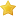• 0 Votes - 0 Average
• 1
• 2
• 3
• 4
• 5
 HARGA H2H CEOBE RELOAD Author Message
IVAN_PURNAMAJuraganPosts: 20
Joined: Oct 2013
Reputation: 0
Thanks: 0
Given 1 thank(s) in 1 post(s)
 Post: #1HARGA H2H CEOBE RELOAD UPDATE HARGA H2H CEOBE TGL 30-10-2015 TELKOMSEL NASIONAL BTS5 = 5.525 BANK + MKIOS BTS10 = 10.525 BANK + MKIOS BTS20 = 19.900 BANK BTS25 = 24.575 BANK TB20 = 19.675 BANK TB25 = 24.500 BANK TB50 = 48.600 BANK TB100 = 96.100 BANK HTS5 = 10.575 MKIOS HTS10 = 10.575 MKIOS HTS20 = 20.075 MKIOS INDOSAT NASIONAL IB5 = 5.325 BANK IB10 = 5.325 BANK IB20 = 20.000 BANK BI5 = 5.375 SEV BI10 = 10.375 SEV BI25 = 24.850 BANK BI30 = 29.750 BANK BI50 = 49.350 SEV BI100 = 97.900 SEV XL + AXIS NASIONAL XLB5 + AXB5 = 5.550 CHIP XLB10 + AXB10 = 10.300 BANK XLB25 + AXB25 = 24.575 BANK XLB50 + AXB50 = 48.950 BANK XLB100 + AXB100 = 98.100 BANK BXL10 + BAX10 = 10.450 BANK BXL25 + BAX25 = 24.650 BANK BXL50 + BAX50 = 49.100 BANK BXL100 + BAX100 = 98.200 BANK THREE NASIONAL BT5 = 4.975 WEB BT10 = 9.850 WEB BT20 = 19.700 WEB BT30 = 29.575 WEB BT50 = 49.225 WEB BT100 = 98.350 WEB SMARTFREN NASIONAL BSF5 = 4.975 BANK BSF10 = 9.885 BANK BSF20 = 19.700 BANK BSF25 = 24.600 BANK BSF50 = 48.900 BANK BSF100 = 97.250 BANK ESIA NASIONAL BE5 = 4.150 CHIP BE10 = 8.200 CHIP BE25 = 20.500 CHIP BE50 = 41.000 CHIP BE100 = 82.000 CHIP ESIA JABOTABEK EJ5 = 3.850 CHIP EJ10 = 7.700 CHIP EJ25 = 19.250 CHIP EJ50 = 38.500 CHIP EJ100 = 77.000 CHIP TELKOMSEL DATA TF5 = 5.450 CHIP TF10 = 10.450 CHIP TF20 = 20.250 CHIP TF25 = 25.100 CHIP TF50 = 49.000 CHIP TF100 = 97.000 CHIP THREE EXTRA KUOTA TI20 = 2.050 CHIP TI80 = 4.800 CHIP TI300 = 9.300 CHIP TI650 = 19.100 CHIP TI1250 = 30.200 CHIP TI4250 = 77.700 CHIP BOLT B25 = 24.100 B50 = 48.100 B100 = 96.100 B150 = 144.100 B200 = 192.100 TELKOMSEL PAKET SMS ==> CEK PAKET SMS *889# T1SMS : 1350 (All Operator 200 SMS Masa Aktif 1 Hari) T5SMS : 5350 (All Operator 1000 SMS Masa Aktif 5 Hari) YANG BERMINAT, HUBUNGI YM CS KAMI BOS: cobpulsa_cs Nama Server: CeoBe RELOAD ID TTS: 213018e03029 Owner: Ivan Purnama Alamat: Jalan Mangga 1/E-200 Pondok Candra Indah, Waru, Sidoarjo - JATIM No HP: 085105002357 / 085648559969 Pin BB: 2102760B Website H2H: http://www.cobpulsa.com/p/harga-h2h-hth.html
10-30-2015 01:04 PM
 « Next Oldest | Next Newest »

Forum Jump:

User(s) browsing this thread: 1 Guest(s)